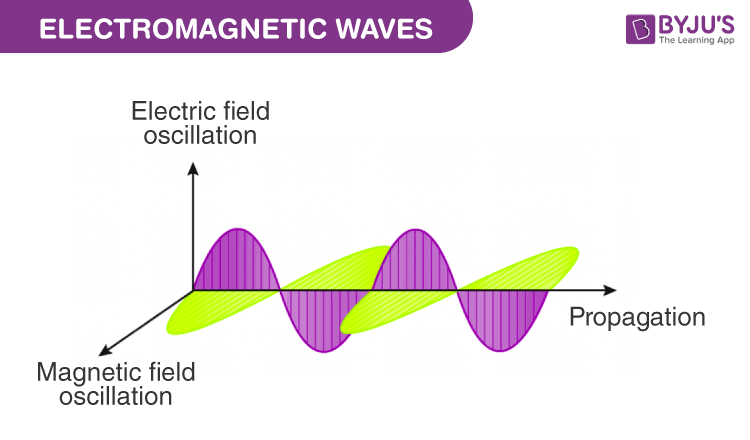# Characteristics Of Electromagnetic Waves

The inherent characteristic of electromagnetic waves is its frequency. According to Maxwell, varying the electric field gives rise to a magnetic field. An accelerated charge produces a time-varying magnetic field which in turn produces a time-varying electric field. Thus, an electromagnetic wave consists of sinusoidal time-varying electric and magnetic fields and both the fields are perpendicular to each other.

You may also want to check out these topics given below!## What are the Characteristics of Electromagnetic Waves?

Listed below are some important characteristics and properties of electromagnetic waves.

• Electromagnetic waves are transverse in nature as they propagate by varying the electric and magnetic fields such that the two fields are perpendicular to each other.
• Accelerated charges are responsible to produce electromagnetic waves.
• Electromagnetic waves have constant velocity in vacuum and it is nearly equal to $$3 × 10^8 m s^{-1}$$ which is denoted by $$C$$ = $$\frac{1}{√{μ_o ϵ_o}}$$.
• Electromagnetic wave propagation does not require any material medium to travel.
• The inherent characteristic of an electromagnetic wave is its frequency. Their frequencies remain unchanged but its wavelength changes when the wave travels from one medium to another.
• The refractive index of a material is given by:$$n$$ = $$√{μ_r ϵ_r}$$
• Electromagnetic wave follows the principle of superposition.
• The light vector (also known as the electric vector) is the reason for the optical effects due to an electromagnetic wave.
• In an electromagnetic wave, the oscillating electric and magnetic fields are in the same phase and their magnitudes have a constant ratio. The ratio of the amplitudes of electric and magnetic fields is equal to the velocity of the electromagnetic wave.$$C$$ = $$\frac{E_0}{B_0}$$
• The energy is carried by the electric and magnetic fields of electromagnetic waves are equal, i.e. the electric energy ($$u_E$$) and the magnetic energy ($$u_M$$) are equal; $$u_E$$ = $$u_M$$.
• There is a vector quantity $$S$$, called the Poynting vector which represents the energy transferred by electromagnetic waves per second per unit area.

$$\overrightarrow{S}$$ = $$\frac{1}{μ}\overrightarrow{E} ~×~ \overrightarrow{B}$$
Electromagnetic radiation from outer space has given us so much information about the universe, its existence and other celestial bodies. Watch interactive videos to visualize electromagnetic waves in more practical ways, download BYJU’S – The Learning App.

Test Your Knowledge On Characteristics Of Em Waves!

#### 1 Comment

1. It’s good to source e-learning as corona hits the world